# Electronics and Communication Engineering - Automatic Control Systems

### Exercise :: Automatic Control Systems - Section 2

16.

The transfer function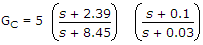can be for

 A. lead compensator B. lag compensator C. lead-lag compensator D. either (a) or (b)

Explanation:

For a lead-lag compensator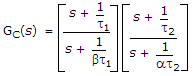β > 1 and a < 1.

17.

The system of the given figure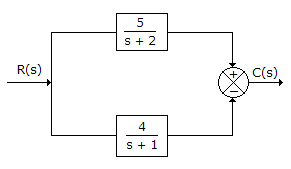A. has two poles and one zero in left half plane B. has two poles and one zero in right half plane C. has two poles in left half plane and zero in right half plane D. none of the above

Explanation:

Since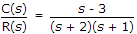there are two poles at s = -2 and s = -1 and one zero at s = +3.

18.

For the given figure C(s)/R(s)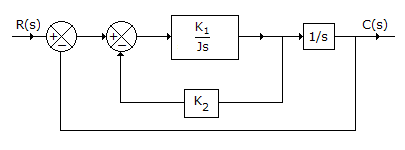A.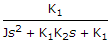B.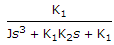C.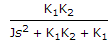D. none of the above

Explanation: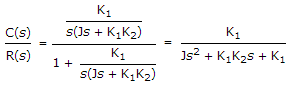.

19.

If phase angle of open loop transfer function becomes - 180° at frequency ω1, then gain margin is equal to

 A. |G(jω1)| B.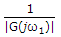C. 1 + |G(jω1)| D.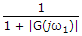Explanation:

If gain is a, gain margin is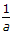. In this case gain is |G(jω1)|.

20.

A system with transfer function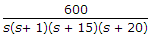may be approximated by the system

 A.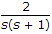B.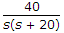C.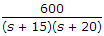D.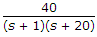Explanation:

Coefficient (s + 15) gives the term e-15t and the coefficient (s + 20) gives the term e-20t.

These can be neglected as compared to terms corresponding to s and (s + 1).